# Percent Composition, Empirical and Molecular FormulasPage 1

#### WATCH ALL SLIDES

Slide 1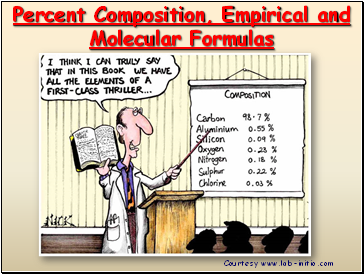Percent Composition, Empirical and Molecular Formulas

Slide 2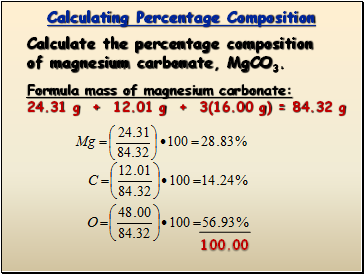## Calculating Percentage Composition

Calculate the percentage composition of magnesium carbonate, MgCO3.

Formula mass of magnesium carbonate:

24.31 g + 12.01 g + 3(16.00 g) = 84.32 g

100.00

Slide 3## Formulas

molecular formula = (empirical formula)n

molecular formula = C6H6 = (CH)6

empirical formula = CH

Empirical formula: the lowest whole number ratio of atoms in a compound.

Molecular formula: the true number of atoms of each element in the formula of a compound.

Slide 4Formulas (continued)

Formulas for ionic compounds are ALWAYS empirical (lowest whole number ratio).

Examples:

NaCl

MgCl2

Al2(SO4)3

K2CO3

Slide 5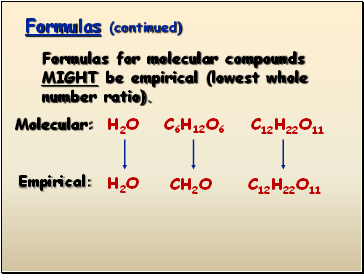Formulas (continued)

Formulas for molecular compounds MIGHT be empirical (lowest whole number ratio).

Molecular:

H2O

C6H12O6

C12H22O11

Empirical:

H2O

CH2O

C12H22O11

Slide 6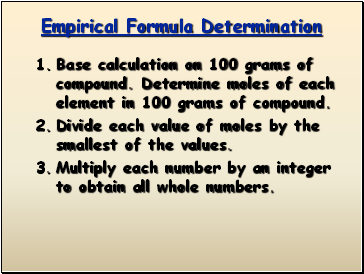## Empirical Formula Determination

Base calculation on 100 grams of compound. Determine moles of each element in 100 grams of compound.

Divide each value of moles by the smallest of the values.

Multiply each number by an integer to obtain all whole numbers.

Slide 7Empirical Formula Determination

Adipic acid contains 49.32% C, 43.84% O, and 6.85% H by mass. What is the empirical formula of adipic acid?

1.Treat % as mass, and convert grams to moles

Slide 8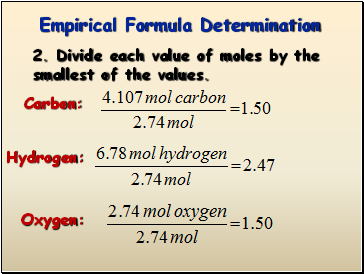Empirical Formula Determination

2. Divide each value of moles by the smallest of the values.

Carbon:

Hydrogen:

Oxygen:

Slide 9Empirical Formula Determination

3. Multiply each number by an integer to obtain all whole numbers.

Carbon: 1.50

Hydrogen: 2.50

Oxygen: 1.00

x 2

x 2

x 2

3

5

2

Empirical formula:

C3H5O2

Slide 10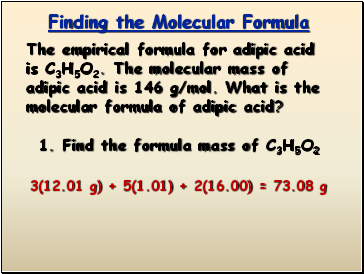## Finding the Molecular Formula

The empirical formula for adipic acid is C3H5O2. The molecular mass of adipic acid is 146 g/mol. What is the molecular formula of adipic acid?

Go to page:
1  2